## Fluorescence Resonance Energy Transfer (FRET)

FRET, also known as Förster Resonance Energy Transfer or just Resonance Energy Transfer (RET), is a long-range non-radiative energy transfer process from an excited donor fluorophore, D, to an acceptor chromophore, A, located within a distance of approximately 1-10 nm. The process can be summarized as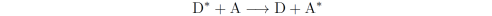where the * denotes an electronically excited state. The rate constant of the energy transfer, kFRET, is a simple function of the distance in between the pair, R, which is why FRET is widely used as a tool for probing molecular interactions and distances between specific sites in macromolecules: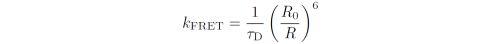Here, τD is the excited state lifetime of D in absence of A and R0 is the critical distance, i.e. the D-A distance at which the FRET efficiency is 50%.

#### FRET efficiency and distance

The FRET efficiency is defined as the quantum yield of the process: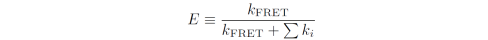where the sum is over all other decay processes but FRET from the excited state of D. Using the relationship between kFRET and τD we get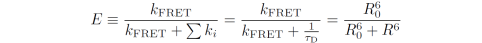Thus, by measuring E experimentally the D-A distance can be calculated as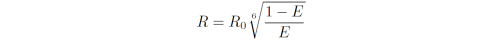provided that the value of R0 is known in advance.

- J. R. Lakowicz, Principles of Fluorescence Spectroscopy, 3rd Ed., Springer, New York, 2006
- B. Valeur, Molecular Fluorescence: Principles and Applications, 2nd Ed., Wiley-VCH, Weinheim, 2012

For a review on advances in quantitative FRET see:

## Measuring Resonance Energy Transfer (FRET) Efficiencies

The FRET efficiency of a D-A pair can be measured experimentally in a number of ways. Following donor excitation the energy transfer is typically determined from one of the following:
1. The D fluorescence intensity
2. The D excited state lifetime
3. The A fluorescence intensity
4. The ratio of D & A fluorescence intensities (ratiometric method)
If A is non-fluorescent (i.e. a dark quencher) E can be determined only from methods 1-2.

#### 1) Measuring energy transfer from donor emission

Ideally, the fluorescence quantum yield (QY) of D is measured for a sample with D+A and for an identical reference sample without A. E is then given by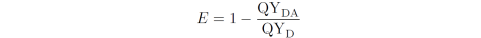Measuring the QY, however, is rarely practical. If the absorbance of D at the excitation wavelength is the exact same in the two samples (e.g. if the exc. wavelengths and concentrations are the same) and the same gain and slit bandwidths are used for the two emission measurements then E can be calculated as: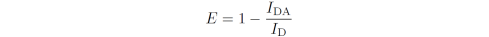where IDA and ID are the total D fluorescence intensities in presence and absence of A, respectively. Since the intensity at a single wavelength is proportional to the total intensity, the intensity values at a wavelength where A does not emit can be used for IDA and ID. Note, however, that single-wavelength readout can be associated with some uncertainty in particular when the wavelength is not at the peak value. If the emission spectra of D and A are greatly overlapping the D component spectrum can be isolated from the mixed spectrum in a|e using spectral decomposition.

The disadvantage of the method above is that the concentration of D is never, or rarely, the exact same throughout different samples (even though you think you made samples of equal concentration). In theory, if the absorbance at the wavelength of excitation is low (A < 0.1 and preferably 0.02-0.07) the concentration difference between the D+A sample and the D-only sample can be accounted for using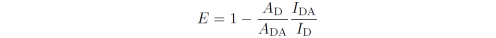where AD and ADA are the D absorbances at the excitation wavelength in the D-only and D+A samples, respectively. In practice, though, obtaining the exact value of ADA can be a very difficult task due to overlapping D and A absorption spectra and often imperfect baselines. Generally speaking, if you use the concentration or absorbance of the sample in order to calculate the FRET efficiency from your data, the measurement is likely inaccurate unless extra care is taken.

#### 2) Measuring energy transfer from donor lifetime

Here the lifetime of D is measured for a sample with D+A and for an identical reference sample without A. E is then calculated as: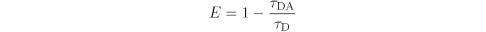Since the lifetime is independent of D concentration, method 2 is less prone to errors introduced due to differences between reference and sample. The disadvantage is that when the donor is characterized by more than one lifetime (and it usually is for FRET dyes attached to biomolecules) there is no simple, universal expression for the FRET efficiency since this will depend on the origin of the different lifetimes measured. A few workaround examples are included here:

2a) If both D-only and D+A displays a multiexponential decay the amplitude-weighted lifetimes of each sample can be used in place of τDA and τD under the assumption of a single D-A distance. The amplitude-weighted lifetime is2b) If the D+A sample has more lifetimes than the D-only sample, or both are highly multi-exponential, the pair is likely characterized by several rather than a single D-A distance. In this case one must turn to more advanced time-resolved or single-molecule methods to characterize the sample. Advanced time-resolved FRET analysis can be performed using DecayFit.

#### 3) Measuring energy transfer from acceptor emission

If A is fluorescent the FRET efficiency can be determined by quantification of the A intensity. This requires either a) an internal reference (i.e. the sample itself) acquired at an excitation wavelength where D does not absorb, or b) an external reference sample without D. In both cases, the absorbance at all excitation wavelengths should be low (A < 0.1 and preferably 0.02-0.07) and the mixed FRET spectrum and reference A spectrum are both acquired using the same gain and slit bandwidths.

In both method 3a and 3b presented below, the intensities can either be obtained as the integrated intensities of the full A emission spectra or as the intensities at a wavelength where D does not emit. Note, however, that D often leaks into most of the A spectrum. Additionally, single-wavelength readout can be associated with some uncertainty in particular when the wavelength is not at the peak value. If the emission spectra of D and A are overlapping the A component spectrum can be isolated from the mixed spectrum in a|e using spectral decomposition.

3a) An internal reference of the A intensity can be obtained by exciting at a wavelength where only A absorbs (i.e. outside the absorption band of D). The FRET efficiency is then determined as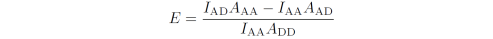where IAD is the A intensity following D excitation, IAA is the A intensity following A excitation, AAA is the A absorbance at the A excitation wavelength, and AAD and ADD are the A and D absorbances, respectively, at the D excitation wavelength. If D and A are present in a 1:1 ratio the FRET efficiency can be calculated based on their extinction coefficients, ε, at the two excitation wavelengths: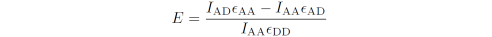This expression takes into account the contribution from direct A excitation at the D wavelength and implicitly takes into account the QY through the use of IAA. Note that this equation assumes that the intensity from the donor does not contribute to the value of IAD, e.g. if IAD was obtained using spectral decompositionThe big advantage is that the reference is measured on the same sample as that used for FRET and the absorbance values are not required. Click here for a derivation of the above equation.

3b) If it is not possible to use direct A excitation outside the absorption band of D, an additional sample without D must be used as reference. In this case the same excitation wavelength is used for both measurements and the FRET efficiency is then calculated aswhere IAD and IA are the intensities of A in presence and absence of D, respectively, AA is the absorbance of A in the A-only sample at the wavelength of excitation, and AAD and AD are the absorbances of A and D, respectively, in the D+A sample. If the concentration of A is the exact same in the two samples, and D and A are present in a 1:1 ratio, then the FRET efficiency can be calculated using their extinction coefficients at the excitation wavelength: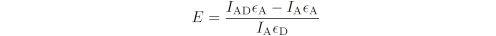The disadvantages of method 3b are the same as for method 1.

#### 4) Measuring energy transfer using donor/acceptor intensity ratio (ratiometric FRET)

If A is fluorescent, FRET is most easily characterized using the ratio between the D and A emission intensities which is known as the ratiometric method. Since the ratio between the D and A intensities depends not only on the value of E but also on the fluorescence quantum yields of the two dyes, ratiometric FRET is usually a relative measure of FRET and should only by used for qualitative purposes or for monitoring relative changes in the FRET efficiency (e.g. kinetic measurements).

In ratiometric FRET, the "relative" FRET efficiency, also known as the proximity ratio, is given by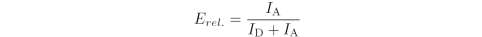where IA and ID are the total A and D fluorescence intensities, respectively, both following D excitation. If the D and A spectra are overlapping the mixed D+A spectrum must be decomposed into the isolated D and A component spectra in order to accurately determine IA and ID.

Going from Erel to an actual FRET efficiency additionally requires at least two correction factors: the contribution from direct A excitation to IA and the ratio between the D and A fluorescence quantum yields. If IA and ID were not determined using spectral decomposition two additional factors must be accounted for, namely the "leakage" of D emission into the value of  IA and leakage of A emission into the value of ID.